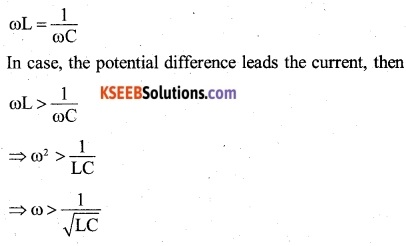# 2nd PUC Physics Question Bank Chapter 6 Electromagnetic Induction

## Karnataka 2nd PUC Physics Question Bank Chapter 6 Electromagnetic Induction

### 2nd PUC Physics Electromagnetic Induction NCERT Text Book Questions and Answers

Question 1.
Predict the direction of induced current in the situations described by the following Figs. 6.18(a) to (f).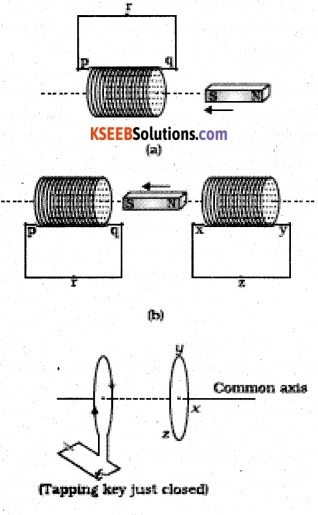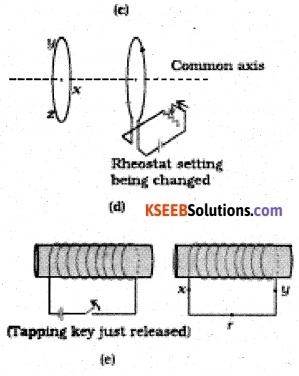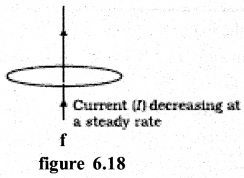(a) According to Lenz’s law, the end of the coil facing the S-pole of the magnet coil become S-pole so as to repel the approaching magnet. For this to happen, the current in the coil will flow in the direction QRPQ.

(b) For the coil on left: For the reason given in (a) the current in the left coil will flow in the direction PRQP.
For the coil on right: According to Lenz’s law, the end of the coil facing the N-pole of the magnet will become S-pole so as to attract the magnet moving away from it. For this to happen, the current in the coil will flow in the direction YZXY.

(c) When the tapping key is just closed, the current flow’s in the coil and hence magnetic flux linked with it starts growing. According to Lenz’s law, the induced current in the adjoining coil should set up in a direction so as to oppose the growth of magnetic flux linked with the coil. It will happen so, if the direction of magnetic field produced by the induced current in the adjoining coil is from right to left i.e. the induced current is produced in the direction YZX.

(d) Due to change in rheostat setting (resistance decreased), the current in the coil and hence magnetic flux linked with it, will start increasing. According to Lenz’s law, the induced current in the adjoining coil should set up in a direction. so as to oppose the increasing in magnetic flux linked with the coil. It will happen. So, if the direction of magnetic field produced by the induced current in the adjoining coil is from right to left i.e. the induced current is produced in the direction ZYX.

(e) Before releasing the tapping keg, the right end of the core is N-pole and the magnetic field linked with the coil is from left to right. As the tapping keg is released, the magnetic field linked with the coil will start collapsing. According to Lenz’s Law the induced current in the adjoining coil should set up in a direction so as to reinforce the collapsing magnetic field. It will happen so, if the direction of magnetic field produced by induced current in the adjoining coil is from left to right i.e. the induced current is produced in the direction XRY.

(f) The current flowing through the straight conductor produces magnetic field in the plane of the coil. Since no magnetic flux is linked with the coil, no induced current will be set up in the coil.Question 2.
Use Lenz’s law to determine the direction of induced current in the situations described by Fig. 6.19:
(a) A wire of irregular shape turning into a circular shape;
(b) A circular loop being deformed into a narrow straight wire.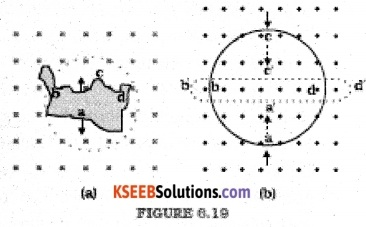In Fig6.19 (a): When a wire of irregular shape turns into a circular (loop the magnetic flux linked with the loop increases due to increase in area) The irregular shape. The induced e.m.f. will cause current to flow in such a direction, so that the wire forming the loop is pulled in ward from all sides. It requires that current should flow.

In figure 6.19 (b): By applying Lenz’s Law, it follows that the current will flow in the direction a, d, c, b, a.

Question 3.
A long solenoid with 15 turns per cm has a small loop of area 2.0 cm2 placed inside the solenoid normal to its axis. If the current carried by the solenoid changes steadily from 2.0 A to 4.0 A in 0.1 s, what is the induced emf in the loop while the current is changing?
The magnetic field produced inside the solenoid,
B = µ0 nl
If A is the area of the loop placed inside the solenoid, then magnetic flux linked with the loop,
Φ = BA = µ0 n IA
If e is the induced e.m.f. produced due to change in current through the solenoid, then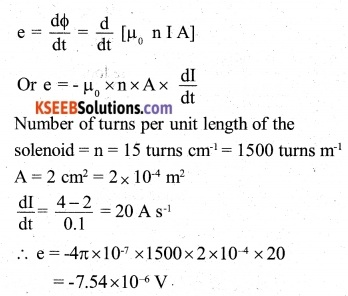Question 4.
A rectangular wire loop of sides 8 cm and 2 cm with a small cut is moving, out of a region of uniform magnetic field of magnitude 0.3 T directed normal to the
loop. What is the emf developed across the cut if the velocity of the loop is 1 cm s-1 in a direction normal to the (a) longer side, (b) shorter side of the loop? For how long does the induced voltage last in each case?Here, B=0.3 T,V=1 cm S-1 10-2 ms-1
In the above expression, 1 is the length of the arm, which is perpendicular to the direction of motion.
(i) Direction of motion normal to longer side: Length of the longer side,
1 = 8 cm = 8 x 10-2 m
∴ e = BlV = 0.3 x 8 x 10-2 ×10-2 = 0.24 x 13-3V
= 0.24 mV
The.e.m.f. will last in the loop, till it does not get out of the magnetic field i.e. for the time the loop takes to travel a distance equal to the length of the shorter arm. If t is the time for which the e.m.f lasts in the loop, then
$$t=\frac { length\quad of\quad the\quad shorter\quad arm }{ V } =\frac { 2\times { 10 }^{ -2 } }{ { 10 }^{ 2 } } =2S$$

(ii) Direction of motion normal to the shorter side:
Length of the shorter side, 1 = 2 cm = 2 x 10-2m
∴ e = Blv = 0.3 x 2 x 102 x 10-2 = 0.06mV
The time for which the e.m.f. lasts in the loop,
$$t=\frac { length\quad of\quad the\quad longer\quad arm }{ V } =\frac { 8\times { 10 }^{ -2 } }{ { 10 }^{ -4 } } =8S$$

Question 5.
A 1.0 m long metallic rod is rotated with an angular frequency of 400 rad s-1 about an axis normal to the rod passing through its one end. The other end of the rod is in contact with a circular metallic ring. A constant and uniform magnetic field of 0.5 T parallel to the axis exists everywhere. Calculate the emf developed between the centre and the ring.
The induced e.m.f. between the center and the circumference of the ring is given by
e = $$\frac { 1 }{ 2 }$$ Bl2 ω[in magnitude]
Here, B = 0.5 T; 1 = tn; ω=400 rad s-1
∴e= $$\frac { 1 }{ 2 }$$ x 0.5 x l2 x 400 = 100V

Question 6.
A circular coil of radius 8.0 cm and 20 turns is rotated about its vertical diameter with an angular speed of 50 rad s_1 in a uniform horizontal magnetic field of magnitude
3.0 x 10-2 Obtain the maximum and average emf induced in the coil. If the coil forms a closed loop of resistance 10 q, calculate the maximum value of current in the coil. Calculate the average power loss due to Joule heating. Where does this power come from?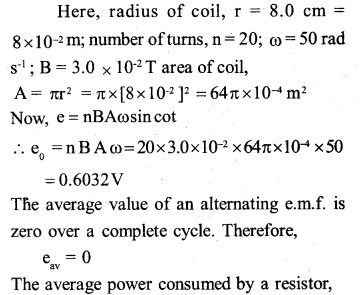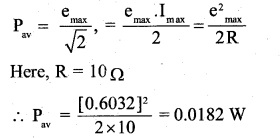The external agent, which keeps the coil rotating, is the source of this power.Question 7.
A horizontal straight wire 10 m long extending from east to west is falling with a speed of 5.0 m s-1, at right angles to the horizontal component of the earth’s ‘ magnetic field, 0.30 × 104 Wbm2.
(a) What is the instantaneous value of the emf induced in the wire?
(b) What is the direction of the emf?
(c) Which end of the wire is at the higher electrical potential?
Here, length of the wire, 1 = 10m;
Velocity of the wire, V = 5.0 ms1
Horizontal component of earth’s magnetic field,
BH = 0.30 x 10-4 Wb m-2
(a) Now,e = BHIv = 0.30 x l0-4x 10 x 5.0
=1.5 x 10-3 V
(b) The induced e.m.f. will be set up from west to east end.
(c) The eastern end will be at higher potential.

Question 8.
Current in a circuit falls from 5.0 A to 0.0 A in 0.1 s. If an average emf of 200 V induced, give an estimate of the self­inductance of the circuit.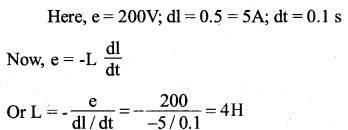Question 9.
A pair of adjacent coils has a mutual inductance of 1.5 H. If the current in one coil changes from 0 to 20 A in 0.5 s, what is the change of flux linkage with the other coil?
Hers, M = 1.5H; dl = 20-0 = 20A;
dt = 0.5 s
Now φ = MI
Or dφ= Mdl=1.5 x 20 = 30Wb.

Question 10.
A jet plane is travelling towards west at a speed of 1800 km/h. What is the voltage difference developed between the ends of the wing having a span of 25 m, if the Earth’s magnetic field at the location has a magnitude of 5 x 10-4 T and the dip angle is 30°?
Here, v = 1,800 Km h-4
= 1,800 x [1,000 m] x [60 x 60 s]-1 = 500 ms-1
Earth’s magnetic field, B = 5.0 x 10-4 t;
angle of dip, 5 = 30°; length of the wing = 25 m
Now, Bv = B sin5 = 5.0x 10-4 sin 30°
= 2.5×10-4T
The vertical component [Bv] of the earth’s field is normal to both the wings and the direction of motion. Therefore, induced e.m.f. produced,
e = Bv x l x V = 2.5 x 500 = 3.125V.

### 2nd PUC Physics Electromagnetic Induction Additional Exercises

Question 11.
Suppose the loop in Exercise 6.4 is stationary but the current feeding the electromagnet that produces the magnetic field is gradually reduced so that the field decreases from its initial value of 0.3 T at the rate of 0.02 Ts-1. If the cut is joined  and the loop has a resistance of 1.6 q, how much power is dissipated by the loop as heat? What is the source of this power?
Here, area of the loop,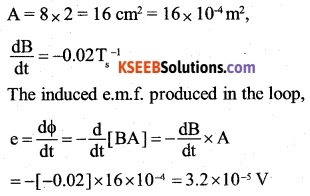Question 12
A square loop of side 12 cm with its sides parallel to X and Y axes is moved with a velocity of 8 cm s-1 in the positive x-direction in an environment containing a magnetic field in the positive Z-direction. The field is neither uniform in space nor constant in time. It has a gradient of 10-3 T cm-1 along the negative x-direction (that is it increases by 103 T cm-1 as one moves in the negative x-direction), and it is decreasing in time at the rate of 10 3 Ts-1. Determine the direction and magnitude of the induced current in the loop if its resistance is 4.50 mΩ.
Here, area of the loop,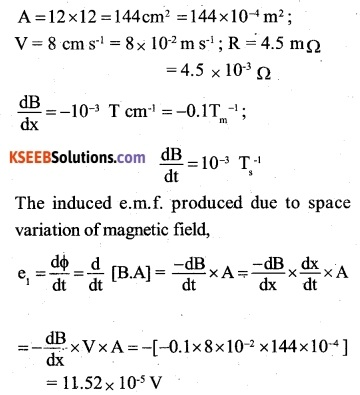The induced e.m.f produced due to space variation of magnetic field.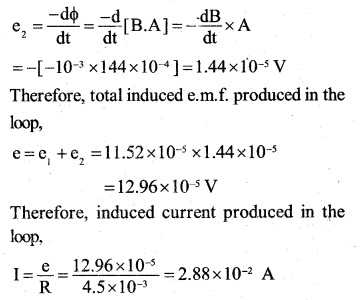According to Lenz’s law, the induced current flows through the loop in a direction so as to cause an increase in the magnetic flux along the positive Z-direction. To an observer, the current will appear to be flowing in anti clock-wise direction, if the loop is moving towards right.

Question 13.
It is desired to measure the magnitude of field between the poles of a powerful loud speaker magnet. A small flat search coil of area 2 cm2 with 25 closely wound turns, is positioned normal to the field direction, and then quickly snatched out of the field region. Equivalently, one can give it a quick 90° turn to bring its plane parallel to the field direction). The total charge flown in the coil (measured by a ballistic galvanometer connected to coil) is 7.5 mC. The combined resistance of the coil and the galvanometer is 0.50 Q. Estimate the field strength of magnet.
Here, number of turns, N = 25
area of coil, A= 2.0 cm2 =2.0 x 10-4 m2
charge flown in the coil, q = 7.5 m
C =7.5 × 10-3 C; resistance of coil R= 0.50 mΩ.
Let B be the strength of the magnetic field, then, magnetic flux linked with the search coil initially.
φ=BAN
When the search coil is brought out of the magnetic field, the magnetic flux linked with the coil finally,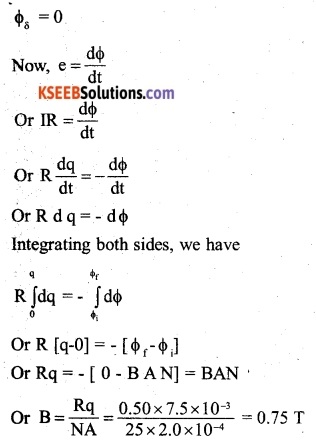Question 14.
Figure 6.20 shows a metal rod PQ resting on the smooth rails AB and positioned between the poles of a permanent magnet. The rails, the rod, and the magnetic field are in three mutual perpendicular directions. A galvanometer G connects the rails through a switch K. Length of the rod = 15 cm, B = 0.50 T, resistance of the closed-loop containing the rod = 9.0 Assume the field to be uniform.

(a) Suppose K is open and the rod is moved with a speed of 12 cm s_1 in the direction shown. Give the polarity and magnitude of the induced emf.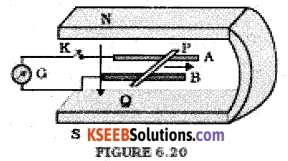(b) Is there an excess charge built up at the ends of the rods when K is open? What if K is closed?

(c) With K open and the rod moving uniformly, there is no net force on the electrons in the rod PQ even though they do experience magnetic force due to the motion of the rod. Explain.

(d) What is the retarding force on the rod when K is closed?

(e) How much power is required (by an external agent) to keep the rod moving at the same speed (=12 cm s-1) when K is closed? How much power is required when K is open?

(f) How much power is dissipated as heat in the closed-circuit? What is the source of this power?

(g) What is the induced emf in the moving rod if the magnetic field is parallel to the rails instead of being perpendicular?
Here, B = 0.50 T; 1 = 15 cm = 15 x 10-2 m;
R = 9.0m Q = 9.0 x 103 fl
(a) Now, e = Bvl
Here, V = 12 cm s-1 = 12 x 10-2 ms-1
∴ e = 0.50 x 12 x 10-2x 15 x 10 2 = 9 x 10-3V
If q is charge on as electron, then the electrons in the rod will experience magnetic Lorentz force $$-q[\vec { v } +\vec { B } ]$$ P. Q. Hence, the end P of the rod will become positive and the end Q will become negative.

(b) When the switch K is open, the electron collect at the end Q. Therefore, excess change is built up at the end Q. However, when the switch K is closed, the accumulated charge at the end Q flows through the circuit,

(c) The magnetic Lorentz force on electron is cancelled by the electronic force acting on it due to the electronic field set up across the two ends due to accumulation of positive and negative charges at the ends P and Q respectively.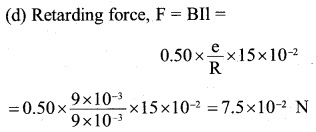(e) When the switch K is closed, power required by the external agent against the retarding force,
P = Fv = 7.5×10-2×12×10-2 = 9×10-3W

(f) Power dissipated as heat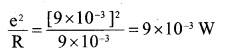The source of this power is the power of external agent against the retarding force,
P = Fv = 7.5×10-2 ×12×10-2= 9×10-3 W

(g) The motion of rod does not cut field lines, hence no induced e.m.f. is produced.Question 15.
An air-cored solenoid with length 30 cm, area of cross-section 25 cm2 and number of turns 500, carries a current of 2.5 A, The current is suddenly switched off in a brief time of 10-3 How much is the average back emf induced across the ends of the open switch in the circuit? Ignore the variation in magnetic field near the ends of the solenoid.
Here, area of cross section of the solenoid,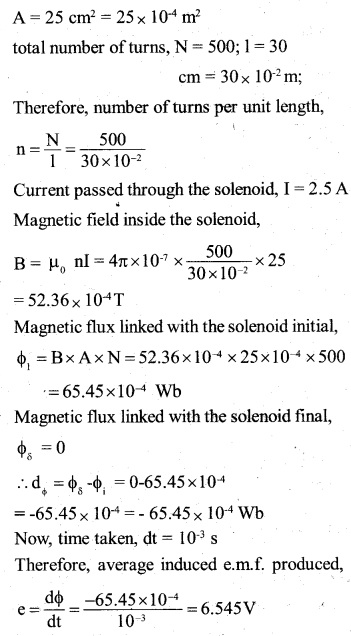Question 16.
(a) Obtain an expression for the mutual inductance between a long straight wire and a square loop of side an as shown in Fig. 6.21.

(b) Now assume that the straight wire carries a current of 50 A and the loop is moved to the right with a constant velocity, v = .10 m/s. Calculate the induced emf in the loop at the instant when x = 0.2 m. Take a = 0.1 m and assume that the loop has a -large resistance.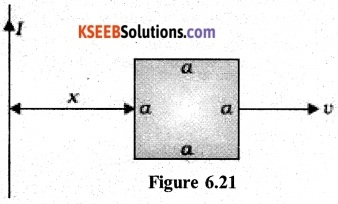(a) Consider as elementary portion of the area of the loop in the form of a strip parallel to the straight wire having a width dx and at a distance x from the wire as shown in the figure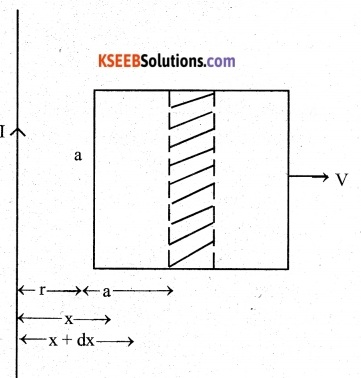If dA is are of this strip, then
dA= a dx
The magnetic field at the strip due to current carrying wire,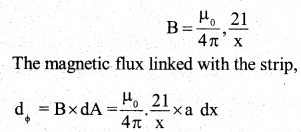The magnetic flux linked with the whole loop can be found by integrating the above between the limits x = r to x = r + b i.e.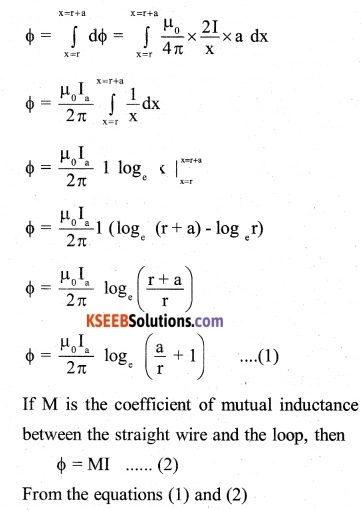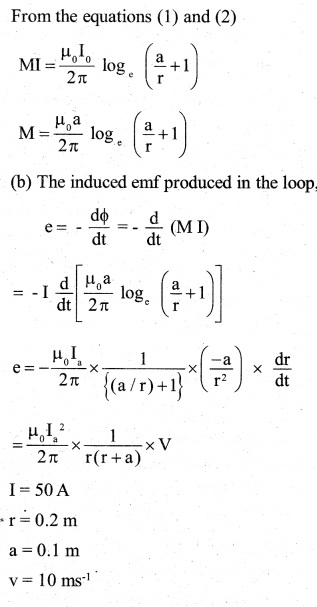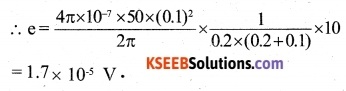Question 17.
A line charge X per unit length is lodged uniformly onto the rim of a wheel of mass M and radius The wheel has light non­conducting spokes and is free to rotate without friction about its axis (Fig. 6.22). A uniform magnetic field extends over a circular region within the rim. It is given by, B = – B0 k (r < a; a < R)= 0 (otherwise)
What is the angular velocity of the wheel after the field is suddenly switched off?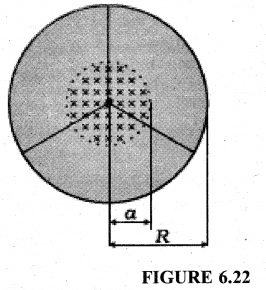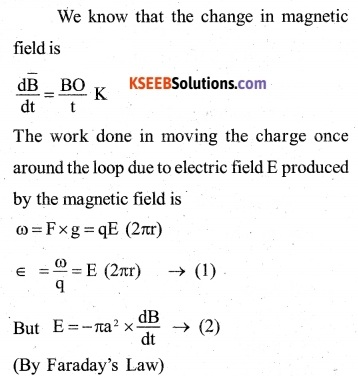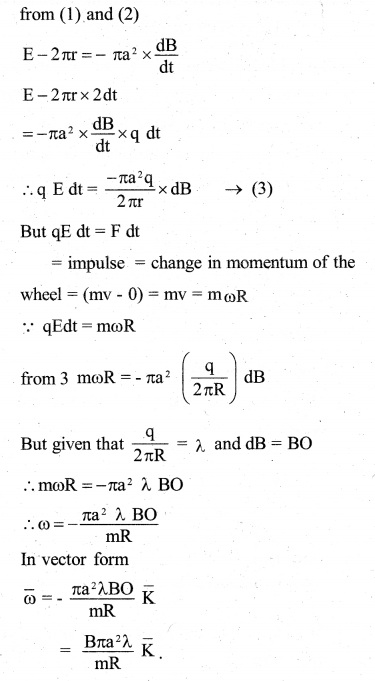Question 1.
Two identical loops, one of copper and another of aluminium are rotated with the same speed in the same magnetic field. In which case, the
(a) induced emf and
(b) induced current will be more and why? (CBSE 2010)
The induced emf will be same in both but the induced current will be more in copper loop as its resistance will be lesser as compared to that of aluminium loop.

Question 2.
A plot of magnetic flux (φ) versus current (I) is shown in figure, for two conductors A and B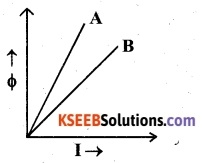Which of the two has a larger value of self-inductance?
From the relation,
φ=LI,
it follows that the self inductance of a conductor is equal to the slope of its graph between φ and I. Therefore, the self inductance of the conductor A has the larger value.

Question 3.
A metal conductor of length lm rotates vertically about one of its ends at angular velocity 5 rad s-1. If the horizontal component of the earth’s magnetic field is
0.2 x 10-4T, then emf developed between the two ends of the conductor is
(A) 5μV
(B) 50μV
(C) 5mV
(D) 50 mV (AIEEE 2004)
The induced emf produced across the two ends of the conductor
e= $$\frac { 1 }{ 2 }$$ Bl2 W=$$\frac { 1 }{ 2 }$$×0.2×10-4×l2 ×5
= 5 ×10-5V = 50 µVQuestion 4.
The self-inductance of the motor of a fan is 10H. In order to impart maximum power at 50Hz, it should be connected to a capacitance of
(A) 4µF
(B) 8µF
(C) 1µF
(D) 2µF (AIEEE 2005)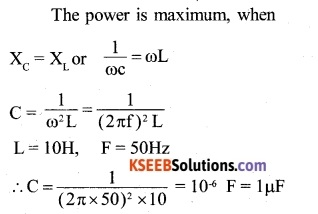Question 5.
Two coils are placed closed to each other. The mutual inductance of the pair of coil depends upon:
(A) The rates at which currents are changing in the two coils
(B) Relative position and orientation of the two coils
(C) The material of wires of the coils
(D) the currents in the two coils
(B) Relative position and orientation of the two coils

Question 6.
A 100 mH coil carries a current of 1A. Energy stored in the form of magnetic field
(A) 0.5J
(B) 1J
(C) 0.05J
(D) 0.1J
L = 100 mH = 0.1. H 1=1 A
The energy stored is given by
U = $$\frac { 1 }{ 2 }$$LI2 = $$\frac { 1 }{ 2 }$$ x 0.1 x l2 = 0.05 J 2    Answer (C).

Question 7.
A straight line conductor of length 0.4 m is moved with a speed of 7ms1 perpendicular to magnetic field of intensity 0.9 Wb nr2. The induced emf across the conductor is.
(A) 1.26 V
(B) 2.52 V
(C) 5.24 V
(D) 25.2 V
B = 0.9 Wb m2
v = 7 ms-1
l = 0.4m
The induced emf produced
φ = BVl = 0.9x 7×0.4
= 2.52 V

Question 8.
What is the power factor of LCR circuit at resonance?
At resonance, the impedance of the circuit is equal to the resistance in the circuit,
i.e. Z = R
∴ power factor of the circuit,
cos φ= $$\frac { R }{ Z } =\frac { R }{ R } =1$$

Question 9.
In a resonant circuit, at which angular frequency, potential difference leads the current?